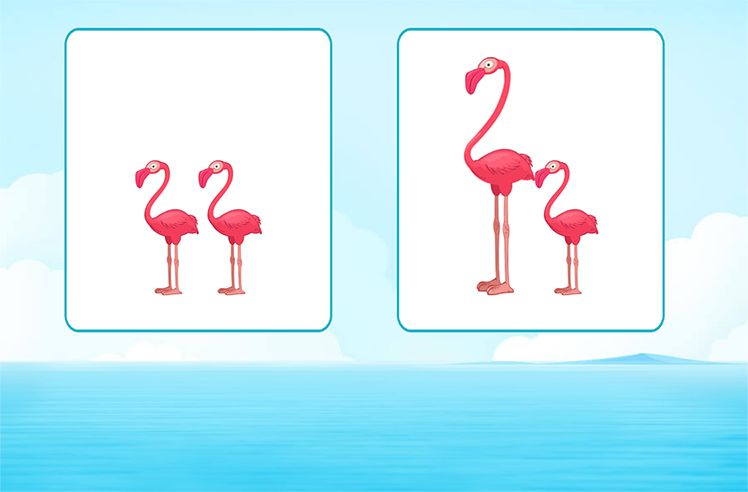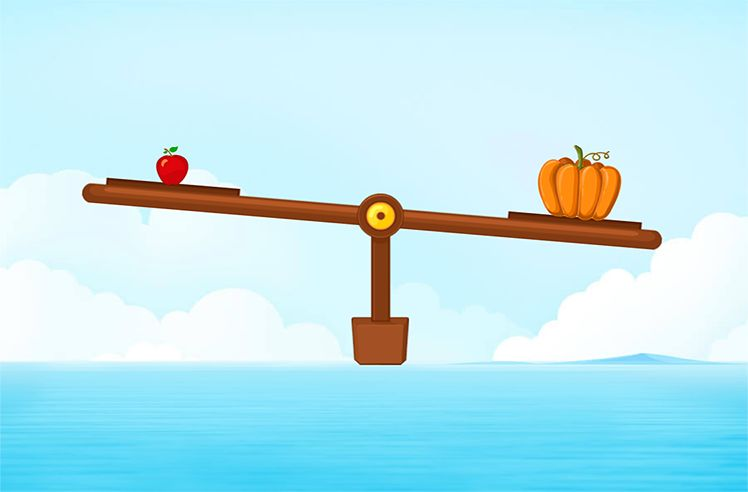# Measure – Definition with Examples

» Measure – Definition with Examples

## Definition of Measure

In math, by measure, we mean quantifying the length, weight, capacity (volume), and many more quantities. Measurement of any quantity is expressed in 2 parts— a numeric value and the specific unit. The following are the most measured quantities:

• Length
• Weight
• Volume
• Time
• Temperature
• Speed

Metric and US Standard are the two main systems of measurement. The table below summarizes the units of measurement used in both scenarios:

## Real-Life Examples of Measuring Different Quantities.

We measure a lot of things in our day-to-day life. A few of those examples are listed below.

1. Measuring the body temperature using a thermometer.
2. Measuring the weight of fruits and vegetables in a shop using a weighing machine.
3. Measuring the height of a child to keep his/her growth record.
4. Measuring time taken by an athlete to complete the race.

Measuring Length

The length of anything tells us how long/short it is. It is measured in units like meters, centimeters, inches, feet, etc. It is measured using various instruments like a ruler, tape measure, meter/yardstick, odometer, screw gauge, etc. A suitable instrument is chosen based on the estimated length of the objects. Some tools measure the length of objects that are very short while some are used to measure the length of very long objects.

Measuring Weight
The weight of anything/anybody tells us about its heaviness. It is measured in units like grams, pounds, kilograms, etc. It is measured using various instruments like weighing scale, body scale, weighing balance, spring balance, etc. Each instrument can hold a specific range of weight. So, for light objects we have different instruments while for very heavy objects we use different instruments.

Measuring Time
Time is measured using a clock. There are two types of clocks— analog and digital.

Analog Clock
An analog clock has numbers from 1 to 12 printed on its dial. It has 3 hands—a second’s hand, a minute hand, and an hour’s hand. The hour hand is the shortest and the slowest while the second’s hand is the thinnest and the fastest. All three hands move around the clock simultaneously at their respective speeds.

Digital Clock
A digital clock shows the time using numerical digits instead of hands-on a dial. Usually, it shows time in 24-hour format. Some digital clocks show time in a 12-hour format as well. They display A.M. or P.M. along with the numeric value. The time before noon is written with the suffix A.M. while the time afternoon and before midnight is written with the suffix P.M.

Measuring Temperature
The temperature of anything tells us how hot or cold it is. It is measured using a device known as a thermometer. It can be measured in 3 different units. That is Celsius, Fahrenheit, and Kelvin.

If you want to know more about Measure and such topics, SplashLearn has a pool of informative resources. SplashLearn provides access to fun activities and puzzles for both parents and students to watch on any device.

## Solved Examples

Example 1: Billy measured the length of the pencil as 7, but forgot to put the unit. Guess what could be the possible unit for the length of the pencil.

Solution: The most suitable unit for measuring the length of a pencil could be inches or centimeters.

So, the measure of Billy’s pencil could be 7 inches or 7 cm.

Example 2: Read the temperature on the given thermometer in celsius.

Solution: For the reading in degrees Celsius, we need to look on the right of the thermometer. The red line ends at the 20-degree mark. So, the temperature shown on this thermometer is 20℃.

Example 3: Which of the following places would be colder?

Florida: 73 ℉ Washington: 50 ℉

Solution: Washington is colder than Florida because its temperature is lesser than the temperature of Florida.

Example 4: Beth says, it is half past 5 in the evening. Which clock shows the correct time?

A –> 5 : 20 A.M.

B –> 5 : 30 A.M.

C –> 5: 30 P.M.

D –> 5 : 45 P.M.

Solution:

Half past 5 means 30 minutes have passed by 5 o’clock. This means the time in digital format will read as 5:30. Also, according to Beth, it is evening, so, the reading should be followed by the suffix P.M. Therefore, it is 5:30 P.M.

Hence, Clock C shows the correct time.

## Practice Problems

1. Length
2. Weight
3. Capacity
4. Temperature
5. Time

The supereon is the largest unit of time.

A light-year is a unit of length. One light-year is the distance that light travels in one year. It is approximately equal to 5.88 trillion miles.Comparing Lengths

Play NowComparing Heights

Play NowComparing Weights

Play Now